# Samacheer Kalvi 10th Maths Solutions Chapter 4 Geometry Ex 4.5

## Tamilnadu Samacheer Kalvi 10th Maths Solutions Chapter 4 Geometry Ex 4.5

Multiple choice questions.
Question 1.
If in triangles ABC and EDF, $$\frac{\mathbf{A B}}{\mathbf{D E}}=\frac{\mathbf{B C}}{\mathbf{F D}}$$ then they will be similar, when
(1) ∠B = ∠E
(2) ∠A = ∠D
(3) ∠B = ∠D
(4) ∠A = ∠F
Solution:
(1) ∠B = ∠E
Hint:Question 2.
In, ∆LMN, ∠L = 60°, ∠M =50° . If ∆LMN ~ ∆PQR then the value of ∠R is
(1) 40°
(2) 70°
(3) 30°
(4) 110°
Solution:
(2) 70°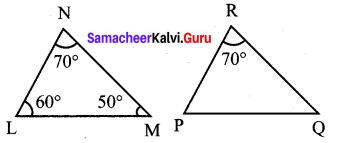∆LMN ~ ∆PQR, ∠R = 70°.

Question 3.
If ∆ABC is an isosceles triangle with ∠C = 90° and AC = 5 cm, then AB is
(1) 2.5 cm
(2) 5 cm
(3) 10 cm
(4) $$5 \sqrt{2}$$ cm
Solution:
(4) $$5 \sqrt{2}$$cm
Hint: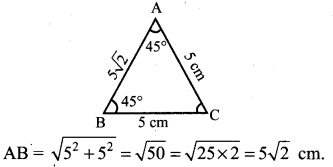Question 4.
In a given figure ST || QR, PS = 2 cm and SQ = 3 cm. Then the ratio of the area of ∆PQR to the area of ∆PST is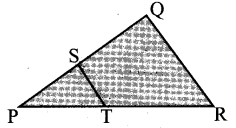(1) 25 : 4
(2) 25 : 7
(3) 25 : 11
(4) 25 : 13
Solution:
(1) 25 : 4
Hint:
Ratio of the area of similar triangles is equal to the ratio of the square of their corresponding sides.
∴ 52 : 22 = 25 : 4

Question 5.
The perimeters of two similar triangles ∆ABC and ∆PQR are 36 cm and 24 cm respectively. If PQ = 10 cm, then the length of AB isSolution:
(4) 15 cm
Hint: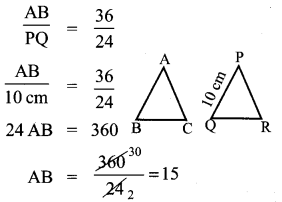Question 6.
If in ∆ABC, DE || BC . AB = 3.6 cm, AC = 2.4 cm and AD = 2.1 cm then the length of AE is
(1) 1.4 cm
(2) 1.8 cm
(3) 1.2 cm
(4) 1.05 cm
Solution:
(1) 1.4 cm$$\frac{3 \cdot 6}{2 \cdot 1}=\frac{2 \cdot 4}{\mathrm{A} \cdot \mathrm{E}}$$
(3.6) (AE) = 2.1 × 2.4
AE = 1.4 cm

Question 7.
In a ∆ABC , AD is the bisector of ∠BAC . If AB = 8 cm, BD = 6 cm and DC = 3 cm. The length of the side AC is
(1) 6 cm
(2) 4 cm
(3) 3 cm
(4) 8 cm
Solution:
(2) 4 cm
Hint: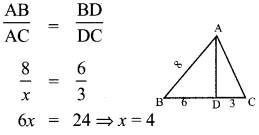Question 8.
In the adjacent figure ∠BAC = 90° and AD ⊥ BC then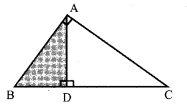(1) BD.CD = BC2
(2) AB.AC = BC2
Solution:Question 9.
Two poles of heights 6 m and 11 m stand vertically on a plane ground. If the distance between their feet is 12 m, what is the distance between their tops?
(1) 13 cm
(2) 14 m
(3.) 15 m
(4) 12.8 m
Solution:
(1) 13 cm
Hint: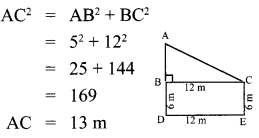Question 10.
In the given figure, PR = 26 cm, QR = 24 cm, PAQ = 90° , PA = 6 cm and QA = 8 cm. Find ∠PQR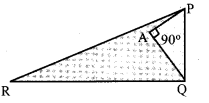(1) 80°
(2) 85°
(3) 75°
(4) 90°
Solution:
(4) 90°
Hint:
PR = 26
QR = 24
∠PAQ = 90°
PQ = 10
PQ = $$\sqrt{26^{2}-24^{2}}=\sqrt{100}$$ = 10
∴ ∠PAQ = 90°Question 11.
A tangent is perpendicular to the radius at the …………..
(1) centre
(2) point of contact
(3) infinity
(4) chord
(2) point of contact

Question 12.
How many tangents can be drawn to the circle from an exterior point?
(1) one
(2) two
(3) infinite
(4) zero
Solution:
(2) two

Question 13.
The two tangents from an external points P to a circle with centre at O are PA and PB. If ∠APB = 70° then the value of ∠AOB is
(1) 100°
(2) 110°
(3) 120°
(4) 130°
Solution:
(2) 110°
Hint: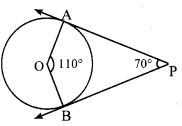Question 14.
In figure CP and CQ are tangents to a circle T with centre at O. ARB is another tangent touching the circle at R. If CP = 11 cm and BC = 7 cm, then the length of BR is
(1) 6 cm
(2) 5 cm
(3) 8 cm
(4) 4 cm
Solution:
(4) 4 cm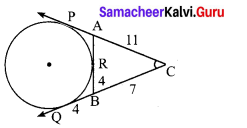BQ = BR
CP = CQ = 11
BC = 7, ∴ BQ = CQ – BC
= 11 – 7 = 4
BR = BQ = 4cmQuestion 15.
In figure if PR is tangent to the circle at P and O is the centre of the circle, then ∠POQ is
(1) 120°
(2) 100°
(3) 110°
(4) 90°
Solution:
(1) 120°
∠POQ = 180° -(30° + 30°)
= 180° – 60°
= 120°